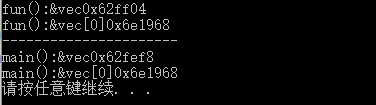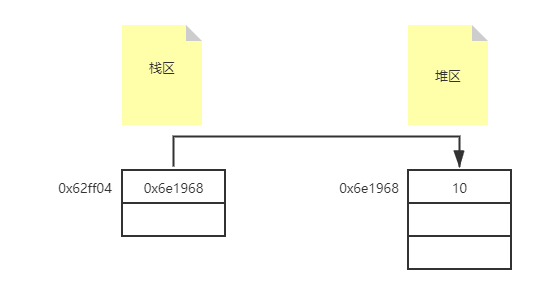# 为什么 C++ 可以返回 Vector 局部变量• vector与动态数组性质相同。

• 具体数据存放在堆区。

• 变量名存放在栈区。

• 变量名中存放的是堆区的首地址。

﻿

C++语言中，所有newmalloc创建的变量均存放在堆区，这已经是一个共识。但是鲜为人知的是，STL库中的容器虽没有经过这两个关键字创建，但同样是存放在堆区。这与动态数组性质相同。如果从汇编角度观察便会发现，容器均调用了allocator来创建。这里便不展开多言。

﻿

﻿

#include <iostream>#include <vector>using namespace std;// 返回一个vector变量，并打印vec的地址和存放在容器中第一个数据的首地址vector<int> fun() {    vector<int> vec;    // 创建vector    vec.push_back(10);   // 添加一个元素    cout << "fun():&vec" << &vec << endl;    cout << "fun():&vec" << &vec << endl;    return vec;   // 返回vector}int main() {    vector<int> vec;   // 创建一个vector    vec = fun();      // 接收传来的参数    cout << "----------------------" <<endl;    cout << "main():&vec" << &vec << endl;    cout << "main():&vec" << &vec << endl;        system("pause");    return 0;}

﻿Fig. 1. 运行现象

﻿Fig. 2. 存放关系示意图

﻿

﻿

﻿

C++本身提供了一个clear()函数，可以清除容器中所有元素。但是此函数并不能释放掉vectorcapacity空间。如果想释放掉所有空间，需使用swap()函数，使用方法如下，若想详细了解释放vector内存，可以参照这位前辈的文章点这里

﻿

vector<int>().swap(vec);   // vec便是需要清空的容器。

﻿

﻿## 评论